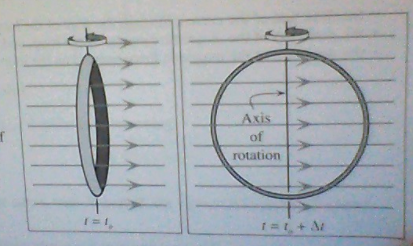# Problem: A copper wire loop is initially at rest in a uniform magnetic field. Between times t = t and t = t, + Δt the loop is rotated about a vertical axis as shown.Will current flow through the wire of the loop during this time interval? If so, indicate the direction of the induced current and explain your reasoning. If not, explain why not.

###### FREE Expert Solution

According to Lenz's law, when a magnetic flux linked with the coil changes, current will be induced in the loop to oppose the change causing it.

Magnetic flux:

$\overline{){{\mathbf{\varphi }}}_{{\mathbf{B}}}{\mathbf{=}}{\mathbf{B}}{\mathbf{A}}{\mathbf{c}}{\mathbf{o}}{\mathbf{s}}{\mathbf{\theta }}}$

In the first case, θ = 0°

83% (355 ratings)###### Problem Details

A copper wire loop is initially at rest in a uniform magnetic field. Between times t = t and t = t, + Δt the loop is rotated about a vertical axis as shown.Will current flow through the wire of the loop during this time interval? If so, indicate the direction of the induced current and explain your reasoning. If not, explain why not.